# 在J2ME/MIDP中实现图像旋转

J2ME概述

J2ME是标准版java（J2SE）面向手机、PDA等各类移动和嵌入式设备的缩减版本，是一种获得众多厂商的支持和广泛使用的移动设备开发平台。图一展示了J2ME技术的体系结构。它分为三层：虚拟机层，配置层，和简表层。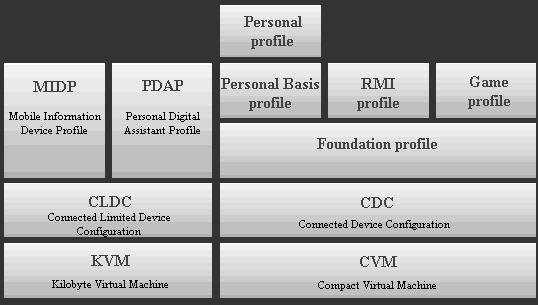图一 J2ME 体系结构

2D旋转的数据基础

 x′ = x * cos θ – y * sin θy′ = y * cos θ + x * sin θ

 x′ = x0 + (x - x0) cos θ - (y - y0) sin θ y′ = y0 + (y - y0) cos θ + (x - x0) sin θ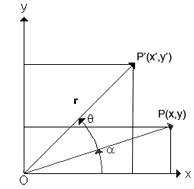一般图像的旋转算法

1、算法思想

 destX = dr + (i - cx) *cos(radian) - (j - cy)*sin(radian);destY = dr + (j - cy) *cos(radian) + (i - cx)*sin(radian);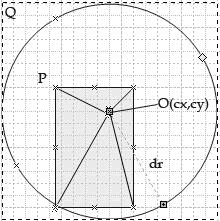图三 旋转算法示意图

2、在J2ME中的算法实现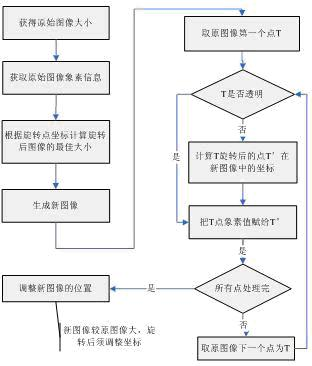图四 算法流程图

 /***@param imgSource 源图像*@param cx 旋转点相对于源图像坐上角横坐标*@param cy 旋转点相对于源图像坐上角纵坐标 *@param theta 图像逆时针旋转的角度*@param dd 含2个元素的整形数组，存放新图像相对源图像沿x轴和y轴的位置偏移量*@return 旋转后的图像**/public Image rotate(Image imgSource, int cx, int cy, double theta, int[] dd) {　if (Math.abs(theta % 360) < 0.1) return imgSource; //角度很小时直接返回　　int w1 = imgSource.getWidth(); //原始图像的高度和宽度　　int h1 = imgSource.getHeight();　　int[] srcMap = new int[w1 * h1];　　imgSource.getRGB(srcMap, 0, w1, 0, 0, w1, h1); //获取原始图像的像素信息　　int dx = cx > w1 / 2 ? cx : w1 - cx; //计算旋转半径　　int dy = cy > h1 / 2 ? cy : h1 - cy;　　double dr = Math.sqrt(dx * dx + dy * dy);　　int wh2 = (int) (2 * dr + 1); //旋转后新图像为正方形，其边长+1是为了防止数组越界　　int[] destMap = new int[wh2 * wh2]; //存放新图像象素的数组　　double destX, destY;　　double radian = theta * Math.PI / 180; //计算角度计算对应的弧度值　　for (int i = 0; i < w1; i++) {　　　for (int j = 0; j < h1; j++) {　　　　if (srcMap[j * w1 + i] >> 24 != 0) { //对非透明点才进行处理　　　　　// 得到当前点经旋转后相对于新图像左上角的坐标　　　　　destX = dr + (i - cx) * Math.cos(radian) + (j - cy)* Math.sin(radian);　　　　　destY = dr + (j - cy) * Math.cos(radian) - (i - cx)* Math.sin(radian); 　　　　　//从源图像中往新图像中填充像素　　　　　destMap[(int) destY * wh2 + (int) destX] = srcMap[j * w1 + i];　　　　}　　　}　　}　　dd = cx-dr; //返回位置偏移分量　　dd = cy-dr;　　return Image.createRGBImage(destMap, wh2, wh2, true); //返回旋转后的图像}

3、旋转失真问题

算法的应用与局限性

1、模拟浮点运算

cldc1.0不支持任何非整形的数值，要实现三角函数的计算，我们可以考虑用已有的整型数来模拟浮点数：把一个整数分成两个域，分别存放浮点的整数和小数部分，这并不难，但要模拟通用的数学函数，如正弦、余弦、二次方根、指数运算等就不那么容易了，需要花费不少时间。由于一些现有的库已经能够很好地完成这些工作，一般情况下，我们可以直接拿来用。

 int xFP = MathFP.toFP(“0.10”);int yFP = MathFP.toFP(“0.2”);int zFP = MathFP.mul(xFP , yFP);System.out.println( MathFP.toString( zFP ) ); //0.02

2、使用预置的三角函数表

 static int[] lookupCosFP = new int;static int[] lookupSinFP = new int;long radianFP ; //用于存放角度的弧度值for(int i = 0; i<360; i++ ) {//将角度转化为弧度，使用MathFP库radianFP=MathFP.div(MathFP.mul(MathFP.toFP(i),MathFP.PI),MathFP.toFP(180)) ;lookupCosFP[i] = MathFP.cos(radianFP); //存入数组lookupSinFP[i] = MathFP.sin(radianFP);}

3、Sprite中的图像旋转

Sprite，即精灵，是在游戏中代表角色的类，它管理所有的图像帧来实现各种动画效果，在游戏开发中有着广泛的应用。如果需要表现动画效果，那用Sprite是再合适不过的了。MIDP2.0中，提供了专门的这样一个类，在构造时只需把图像对象作为参数传递。Sprite类自身提供了图像反射和成90度整数倍旋转的功能。如果要实现任意角度旋转，本质上跟上面的Image的旋转没有分别，只是在Sprite中内置了精灵的位置等信息，管理起来会更加方便高效。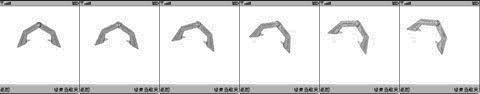图五 钩子的旋转效果

4、局限性

(1)该算法的使用过程中生成了较大的图像，比较适合于图像绕固定点连续旋转情况。如果实际中图像只需做一次旋转，或旋转点经常变换，这种方法会产生较大的无效区域，增加处理的负担，此时，旋转后图像的大小最好根据旋转点和角度做最优化计算。

(2)算法需要获得图像的象素信息，这在midp2.0才给予支持，如果要在midp1.0的机器上实现图像旋转，须借助于设备厂商专用开发包，如Nokia开发包就提供了DirectUtils类实现类似上面createRGBImage()的功能。当然这只能在相应设备上才能用。

(3)算法要求设备支持Alpha通道，否则不能正常的表现效果。

(4)基于该方法的Sprite对象在做碰撞检测时，须采用象素检测的方法。

1、预置图像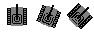2、使用TinyLine 2D

J2ME作为移动信息设备上的开发应用程序的开放平台，获得了众多厂商的支持，和越来越广泛的使用。本文从数学的基础出发，提出了实现图像按任意角度旋转的一种方案并给出了基于J2ME/MIDP平台的实现，给出了局限性分析，最后引出了实现图像旋转的另外两种参考性方法。其实方法并没有好劣之分，只有适合不适合之说，在实际应用中，我们应根据具体的需求，选择最合适的方案。希望本文能给读者在J2ME开发中需要用到图像旋转的功能时提供有益的参考。

 版权所有：UML软件工程组织